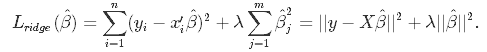Discussion Forum | Board Infinity# What are Lasso and Ridge regression?

Lasso and Ridge regression

In Ridge Regression, the OLS loss function is augmented in such a way that we not only minimize the sum of squared residuals but also penalize the size of parameter estimates, in order to shrink them towards zero:Solving this for
β^ β^ gives the ridge regression estimates
β^ ridge=(X′ X+λI)−1(X′Y)
β^ridge=(X′X+λI)−1(X′Y), where I denote the identity matrix.
The λ parameter is the regularization penalty.
Ridge regression assumes the predictors are standardized and the response is centered